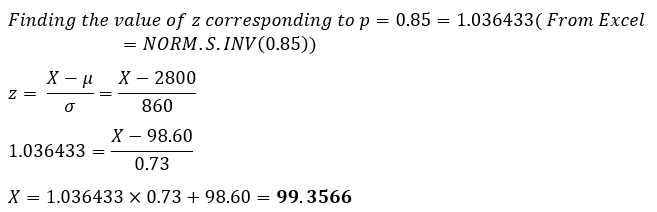# The body temperatures of adults are normally distributed with a mean of 98.60 and a standard deviation of 0.73. What temperature represents the 85th percentile?

Question
14 views

The body temperatures of adults are normally distributed with a mean of 98.60 and a standard deviation of 0.73. What temperature represents the 85th percentile?

check_circle

Step 1

Given data

Mean = 98.60

Standard deviation = 0.73

Probability = 0.85...

### Want to see the full answer?

See Solution

#### Want to see this answer and more?

Solutions are written by subject experts who are available 24/7. Questions are typically answered within 1 hour.*

See Solution
*Response times may vary by subject and question.
Tagged in